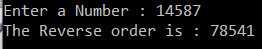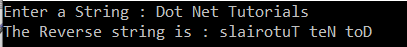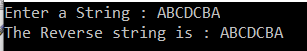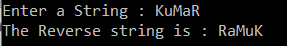# Reverse Number Program in C#

## Reverse a Number and a String in C# with Examples

In this article, I am going to discuss the How to Reverse a Number and a String in C# with Examples. Please read our previous article where we discussed the Palindrome Program in C# with some examples. This is one of the most frequently asked written interview questions in C#. As part of this article, we are going to discuss the following pointers.

1. How to Reverse a Given Number in C#?
2. How to Reverse a Given String in C#?
##### How to reverse a given number in C#?

In the following C# program, we take the input number from the console and then reverse that number.

```using System;
namespace LogicalPrograms
{
public class Program
{
static void Main(string[] args)
{
Console.Write("Enter a Number : ");
int reminder, reverse = 0;

while (number > 0)
{
//Get the remainder by dividing the number with 10
reminder = number % 10;

//multiply the sum with 10 and then add the reminder
reverse = (reverse * 10) + reminder;

//Get the quotient by dividing the number with 10
number = number / 10;
}

Console.WriteLine(\$"The Reverse order is : {reverse}");
}
}
}```
###### Output:##### How to reverse a string in C#?

In the following C# program, we take the string as an input from the console. Then we reverse the string using for loop.

```using System;
namespace LogicalPrograms
{
public class Program
{
static void Main(string[] args)
{
Console.Write("Enter a String : ");
string reverse = string.Empty;

for (int i = name.Length - 1; i >= 0; i--)
{
reverse += name[i];
}

Console.WriteLine(\$"The Reverse string is : {reverse}");
}
}
}
```
###### Output:##### Reverse a string Using Foreach loop in C#:

Let us see how to reverse a string using for each loop in C#.

```using System;
namespace LogicalPrograms
{
public class Program
{
static void Main(string[] args)
{
Console.Write("Enter a String : ");
string reverse = string.Empty;
foreach (char c in name)
{
reverse = c + reverse;
}

Console.WriteLine(\$"The Reverse string is : {reverse}");
}
}
}
```
###### Output:##### Reverse a string using Array.Reverse Method in C#:

In the following example, we take a string as an input from the console and then convert that string to a character array. Then we use the Array class Reverse method to reverse the elements of the character array. Once we reverse the elements of the character array, then we create a string from that character array.

```using System;
namespace LogicalPrograms
{
public class Program
{
static void Main(string[] args)
{
Console.Write("Enter a String : ");

char[] nameArray = name.ToCharArray();
Array.Reverse(nameArray);
string reverse = new string(nameArray);

Console.WriteLine(\$"The Reverse string is : {reverse}");